Open in App
Not now

# Linear Search Algorithm

• Difficulty Level : Basic
• Last Updated : 27 Mar, 2023

Linear Search is defined as a sequential search algorithm that starts at one end and goes through each element of a list until the desired element is found, otherwise the search continues till the end of the data set.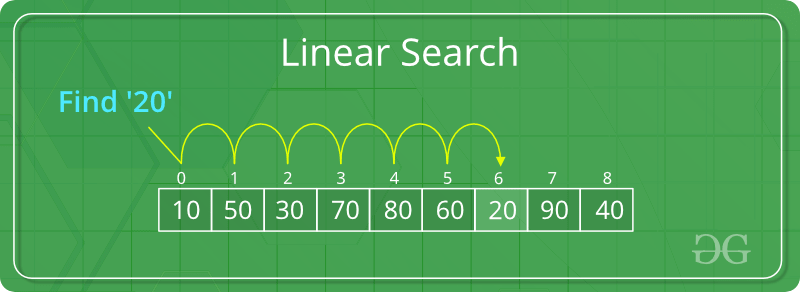Example of Linear Search

## How Linear Search Works?

• Step 1: First, read the search element (Target element) in the array.
• Step 2: Set an integer i = 0 and repeat steps 3 to 4 till i reaches the end of the array.
• Step 3: Match the key with arr[i].
• Step 4: If the key matches, return the index. Otherwise, increment i by 1.

### Illustration of Linear Search:

Consider the array arr[] = {10, 50, 30, 70, 80, 20, 90, 40} and key = 20

Step 1: Set i = 0 and check key with arr.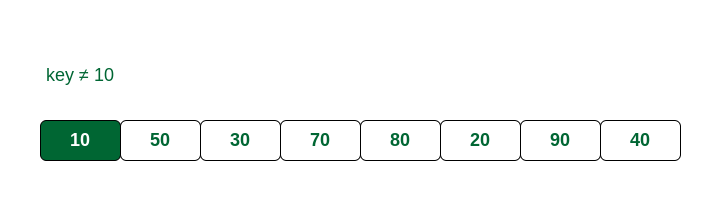Compare key with index 0

Step 2: key and arr are not the same. So make i = 1 and match key with arr.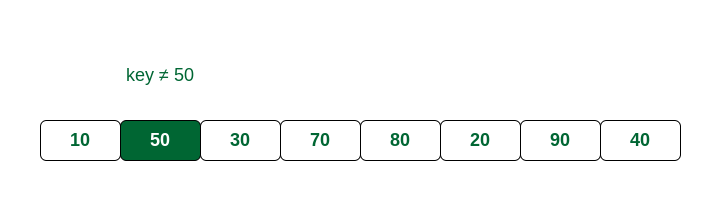Compare key with index 1

Step 3: arr and key are different. Increment i and compare key with arr.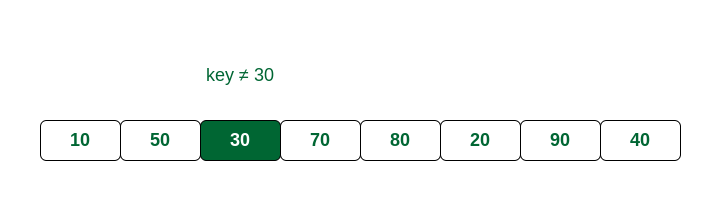Compare key with index 2

Step 4: arr is not the same with key. Increment i and compare key with arr.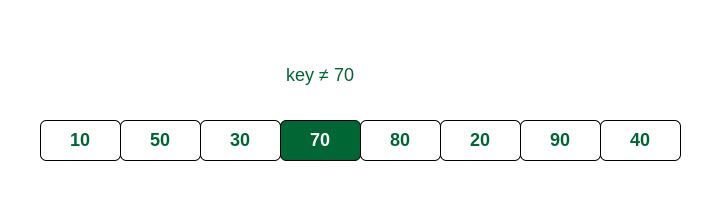Compare key with index 3

Step 5: key and arr are different. Make i = 4 and compare key with arr.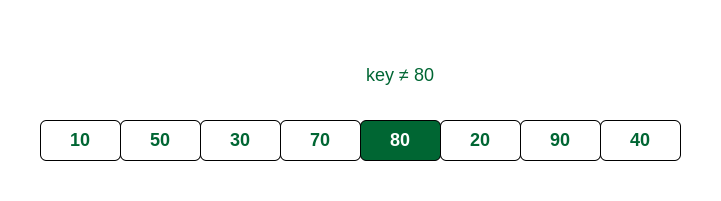Compare key with index 4

Step 6: key and arr are not same. Make i = 5 and match key with arr.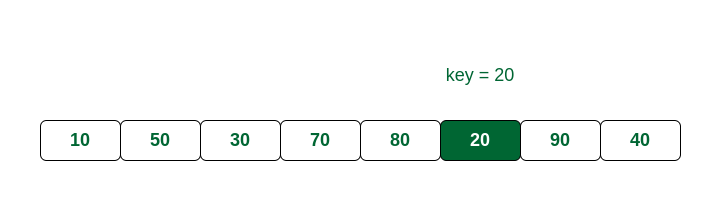Compare key with index 5

We can see here that key is present at index 5.

Recommended Problem

## Complexity Analysis of Linear Search:

Time Complexity:

• Best Case: In the best case, the key might be present at the first index. So the best case complexity is O(1)
• Worst Case: In the worst case, the key might be present at the last index i.e., opposite to the end from which the search has started in the list. So the worst case complexity is O(N) where N is the size of the list.
• Average Case: O(N)

Auxiliary Space: O(1) as except the variable to iterate through the list, no other variable is used.

### Iterative implementation of Linear Search:

Below is the implementation of the linear search algorithm:

## C

 `// C code to linearly search x in arr[]. If x` `// is present then return its location, otherwise` `// return -1`   `#include `   `int` `search(``int` `arr[], ``int` `N, ``int` `x)` `{` `    ``int` `i;` `    ``for` `(i = 0; i < N; i++)` `        ``if` `(arr[i] == x)` `            ``return` `i;` `    ``return` `-1;` `}`   `// Driver code` `int` `main(``void``)` `{` `    ``int` `arr[] = { 2, 3, 4, 10, 40 };` `    ``int` `x = 10;` `    ``int` `N = ``sizeof``(arr) / ``sizeof``(arr);`   `    ``// Function call` `    ``int` `result = search(arr, N, x);` `    ``(result == -1)` `        ``? ``printf``(``"Element is not present in array"``)` `        ``: ``printf``(``"Element is present at index %d"``, result);` `    ``return` `0;` `}`

## C++

 `// C++ code to linearly search x in arr[]. If x` `// is present then return its location, otherwise` `// return -1`   `#include ` `using` `namespace` `std;`   `int` `search(``int` `arr[], ``int` `N, ``int` `x)` `{` `    ``int` `i;` `    ``for` `(i = 0; i < N; i++)` `        ``if` `(arr[i] == x)` `            ``return` `i;` `    ``return` `-1;` `}`   `// Driver code` `int` `main(``void``)` `{` `    ``int` `arr[] = { 2, 3, 4, 10, 40 };` `    ``int` `x = 10;` `    ``int` `N = ``sizeof``(arr) / ``sizeof``(arr);`   `    ``// Function call` `    ``int` `result = search(arr, N, x);` `    ``(result == -1)` `        ``? cout << ``"Element is not present in array"` `        ``: cout << ``"Element is present at index "` `<< result;` `    ``return` `0;` `}`

## Java

 `// Java code for linearly searching x in arr[]. If x` `// is present then return its location, otherwise` `// return -1`   `class` `GFG {` `    ``public` `static` `int` `search(``int` `arr[], ``int` `x)` `    ``{` `        ``int` `N = arr.length;` `        ``for` `(``int` `i = ``0``; i < N; i++) {` `            ``if` `(arr[i] == x)` `                ``return` `i;` `        ``}` `        ``return` `-``1``;` `    ``}`   `    ``// Driver code` `    ``public` `static` `void` `main(String args[])` `    ``{` `        ``int` `arr[] = { ``2``, ``3``, ``4``, ``10``, ``40` `};` `        ``int` `x = ``10``;`   `        ``// Function call` `        ``int` `result = search(arr, x);` `        ``if` `(result == -``1``)` `            ``System.out.print(` `                ``"Element is not present in array"``);` `        ``else` `            ``System.out.print(``"Element is present at index "` `                             ``+ result);` `    ``}` `}`

## Python3

 `# Python3 code to linearly search x in arr[].` `# If x is present then return its location,` `# otherwise return -1`     `def` `search(arr, N, x):`   `    ``for` `i ``in` `range``(``0``, N):` `        ``if` `(arr[i] ``=``=` `x):` `            ``return` `i` `    ``return` `-``1`     `# Driver Code` `if` `__name__ ``=``=` `"__main__"``:` `    ``arr ``=` `[``2``, ``3``, ``4``, ``10``, ``40``]` `    ``x ``=` `10` `    ``N ``=` `len``(arr)`   `    ``# Function call` `    ``result ``=` `search(arr, N, x)` `    ``if``(result ``=``=` `-``1``):` `        ``print``(``"Element is not present in array"``)` `    ``else``:` `        ``print``(``"Element is present at index"``, result)`

## C#

 `// C# code to linearly search x in arr[]. If x` `// is present then return its location, otherwise` `// return -1` `using` `System;`   `class` `GFG {` `    ``public` `static` `int` `search(``int``[] arr, ``int` `x)` `    ``{` `        ``int` `N = arr.Length;` `        ``for` `(``int` `i = 0; i < N; i++) {` `            ``if` `(arr[i] == x)` `                ``return` `i;` `        ``}` `        ``return` `-1;` `    ``}`   `    ``// Driver's code` `    ``public` `static` `void` `Main()` `    ``{` `        ``int``[] arr = { 2, 3, 4, 10, 40 };` `        ``int` `x = 10;`   `        ``// Function call` `        ``int` `result = search(arr, x);` `        ``if` `(result == -1)` `            ``Console.WriteLine(` `                ``"Element is not present in array"``);` `        ``else` `            ``Console.WriteLine(``"Element is present at index "` `                              ``+ result);` `    ``}` `}`   `// This code is contributed by DrRoot_`

## PHP

 ``

## Javascript

 ``

Output

`Element is present at index 3`

Time complexity: O(N)
Auxiliary Space: O(1)

### Recursive Approach for Linear Search:

We can also utilize linear search using a recursive function. In this case, the iteration is done using a recursion.

Follow the given steps to solve the problem:

• If the size of the array is zero then, return -1, representing that the element is not found. This can also be treated as the base condition of a recursion call.
• Otherwise, check if the element at the current index in the array is equal to the key or not i.e, arr[size – 1] == key
• If equal, then return the index of the found key.

Below is the implementation of the above approach:

## C

 `#include `   `// Define a function to perform the linear search` `int` `linearSearch(``int` `arr[], ``int` `size, ``int` `key)` `{` `    ``// If the size of the array is zero, return -1` `    ``if` `(size == 0) {` `        ``return` `-1;` `    ``}`   `    ``// Check if the element at the current index` `    ``// is equal to the key` `    ``if` `(arr[size - 1] == key) {` `        ``// If equal, return the index` `        ``return` `size - 1;` `    ``}` `    ``else` `{` `        ``// If not equal, call the function again` `        ``// with the size reduced by 1` `        ``return` `linearSearch(arr, size - 1, key);` `    ``}` `}`   `// Driver code` `int` `main()` `{` `    ``int` `arr[] = { 5, 15, 6, 9, 4 };` `    ``int` `key = 4;` `    ``int` `index` `        ``= linearSearch(arr, ``sizeof``(arr) / ``sizeof``(``int``), key);` `    ``if` `(index == -1) {` `        ``printf``(``"Key not found in the array.\n"``);` `    ``}` `    ``else` `{` `        ``printf``(``"The element %d is found at %d index of the "` `               ``"given array \n"``,` `               ``key, index);` `    ``}` `    ``return` `0;` `}`

## C++14

 `// C++ Recursive Code For Linear Search` `#include ` `using` `namespace` `std;`   `int` `linearsearch(``int` `arr[], ``int` `size, ``int` `key)` `{` `    ``if` `(size == 0) {` `        ``return` `-1;` `    ``}` `    ``else` `if` `(arr[size - 1] == key) {` `        ``// Return the index of found key.` `        ``return` `size - 1;` `    ``}` `    ``else` `{` `        ``int` `ans = linearsearch(arr, size - 1, key);` `        ``return` `ans;` `    ``}` `}`   `// Driver Code` `int` `main()` `{` `    ``int` `arr = { 5, 15, 6, 9, 4 };` `    ``int` `key = 4;`   `    ``// Function call` `    ``int` `ans = linearsearch(arr, 5, key);` `    ``if` `(ans == -1) {` `        ``cout << ``"The element "` `<< key << ``" is not found."` `             ``<< endl;` `    ``}` `    ``else` `{` `        ``cout << ``"The element "` `<< key << ``" is found at "` `             ``<< ans << ``" index of the given array."` `<< endl;` `    ``}` `    ``return` `0;` `}` `// Code contributed by pragatikohli`

## Java

 `// Java Recursive Code For Linear Search` `import` `java.io.*;`   `class` `Test {` `    ``static` `int` `arr[] = { ``5``, ``15``, ``6``, ``9``, ``4` `};`   `    ``// Recursive Method to search key in the array` `    ``static` `int` `linearsearch(``int` `arr[], ``int` `size, ``int` `key)` `    ``{` `        ``if` `(size == ``0``) {` `            ``return` `-``1``;` `        ``}` `        ``else` `if` `(arr[size - ``1``] == key) {`   `            ``// Return the index of found key.` `            ``return` `size - ``1``;` `        ``}` `        ``else` `{` `            ``return` `linearsearch(arr, size - ``1``, key);` `        ``}` `    ``}`   `    ``// Driver method` `    ``public` `static` `void` `main(String[] args)` `    ``{` `        ``int` `key = ``4``;`   `        ``// Function call to find key` `        ``int` `index = linearsearch(arr, arr.length, key);` `        ``if` `(index != -``1``)` `            ``System.out.println(` `                ``"The element "` `+ key + ``" is found at "` `                ``+ index + ``" index of the given array."``);`   `        ``else` `            ``System.out.println(``"The element "` `+ key` `                               ``+ ``" is not found."``);` `    ``}` `}`   `// This Code is submitted by Susobhan Akhuli`

## Python3

 `# Python Program to Implement Linear Search Recursively`     `def` `linear_search(arr, key, size):`   `    ``# If the array is empty we will return -1` `    ``if` `(size ``=``=` `0``):` `        ``return` `-``1`   `    ``elif` `(arr[size ``-` `1``] ``=``=` `key):`   `        ``# Return the index of found key.` `        ``return` `size ``-` `1` `    ``else``:` `        ``return` `linear_search(arr, key, size ``-` `1``)`     `# Driver code` `if` `__name__ ``=``=` `"__main__"``:` `    ``arr ``=` `[``5``, ``15``, ``6``, ``9``, ``4``]` `    ``key ``=` `4` `    ``size ``=` `len``(arr)`   `    ``# Calling the Function` `    ``ans ``=` `linear_search(arr, key, size)` `    ``if` `ans !``=` `-``1``:` `        ``print``(``"The element"``, key, ``"is found at"``,` `              ``ans, ``"index of the given array."``)` `    ``else``:` `        ``print``(``"The element"``, key, ``"is not found."``)`   `# Code Contributed By - DwaipayanBandyopadhyay` `# Code is modified by Susobhan Akhuli`

## C#

 `// C# Recursive Code For Linear Search` `using` `System;`   `static` `class` `Test {` `    ``static` `int``[] arr = { 5, 15, 6, 9, 4 };`   `    ``// Recursive Method to search key in the array` `    ``static` `int` `linearsearch(``int``[] arr, ``int` `size, ``int` `key)` `    ``{` `        ``if` `(size == 0) {` `            ``return` `-1;` `        ``}` `        ``else` `if` `(arr[size - 1] == key) {`   `            ``// Return the index of found key.` `            ``return` `size - 1;` `        ``}` `        ``else` `{` `            ``return` `linearsearch(arr, size - 1, key);` `        ``}` `    ``}`   `    ``// Driver method` `    ``public` `static` `void` `Main(String[] args)` `    ``{` `        ``int` `key = 4;`   `        ``// Method call to find key` `        ``int` `index = linearsearch(arr, arr.Length, key);`   `        ``if` `(index != -1)` `            ``Console.Write(``"The element "` `+ key` `                          ``+ ``" is found at "` `+ index` `                          ``+ ``" index of the given array."``);` `        ``else` `            ``Console.Write(``"The element "` `+ key` `                          ``+ ``" is not found."``);` `    ``}` `}`   `// This Code is submitted by Susobhan Akhuli`

## PHP

 ``

## Javascript

 `// JavaScript Recursive Code For Linear Search`   `let linearsearch = (arr, size, key) => {` `  ``if` `(size == 0) {` `    ``return` `-1;` `  ``}` `  ``else` `if` `(arr[size - 1] == key)` `  ``{` `  `  `    ``// Return the index of found key.` `    ``return` `size - 1;` `  ``}` `  ``else` `  ``{` `    ``let ans = linearsearch(arr, size - 1, key);` `    ``return` `ans;` `  ``}` `};`   `// Driver Code` `let main = () => {` `  ``let arr = [5, 15, 6, 9, 4];` `  ``let key = 4;` `  ``let ans = linearsearch(arr, 5, key);` `  ``if` `(ans == -1) {` `    ``console.log(`The element \${key} is not found.`);` `  ``} ``else` `{` `    ``console.log(` `      ```The element \${key} is found at \${ans} index of the given array.`` `    ``);` `  ``}` `  ``return` `0;` `};`   `main();`   `// This code is contributed by Aman Singla...`

Output

`The element 4 is found at 4 index of the given array.`

Time Complexity: O(N)
Auxiliary Space: O(N), for using recursive stack space.

• Linear search is simple to implement and easy to understand.
• Linear search can be used irrespective of whether the array is sorted or not. It can be used on arrays of any data type.
• Does not require any additional memory.
• It is a well suited algorithm for small datasets.

## Drawbacks of Linear Search:

• Linear search has a time complexity of O(n), which in turn makes it slow for large datasets.
• Not suitable for large arrays.
• Linear search can be less efficient than other algorithms, such as hash tables.

## Improving Linear Search:

As seen above, the time taken to search an element using linear search is linear. This is a drawback for using linear search for large data sets. It is observed that when searching for a key element, then there is a possibility for searching the same key element again and again. We can improve the performance of linear search using this observation.

The goal is that if the same element is searched again then the operation must take lesser time. Therefore, in such a case, Linear Search can be improved by using the following two methods:

• Transposition
• Move to Front

### Sentinel Linear Search:

Another improved version of the linear search is the Sentinel Linear Search. In this approach, the target is to reduce the number of comparisons performed to search an element. To read more about it, refer to the article on “Sentinel Linear Search“.

## When to use Linear Search?

• When we are dealing with a small dataset.
• When you need to find an exact value.
• When you are searching a dataset stored in contiguous memory.
• When you want to implement a simple algorithm.

## Conclusion:

To conclude, it can be said that linear search is a simple and flexible algorithm for finding whether an element is present within an array. It sequentially examines each element of the array and is better for being used in unsorted data sets.

My Personal Notes arrow_drop_up
Related Articles